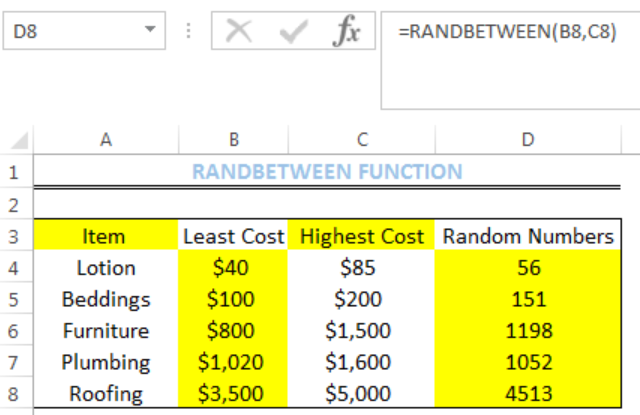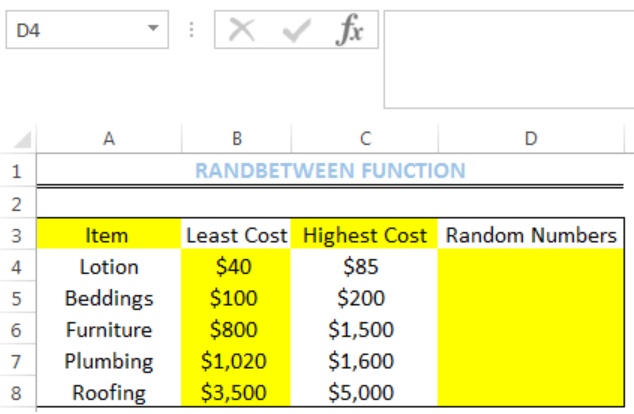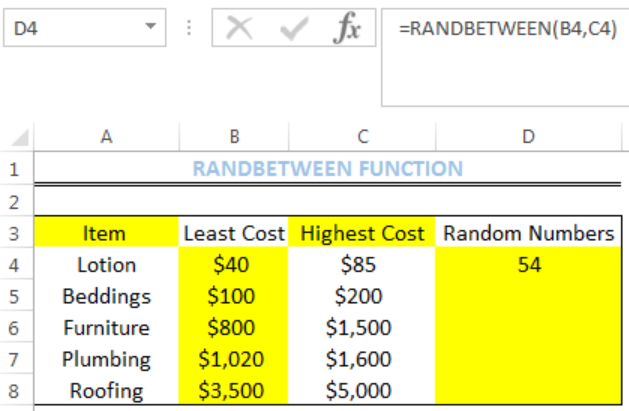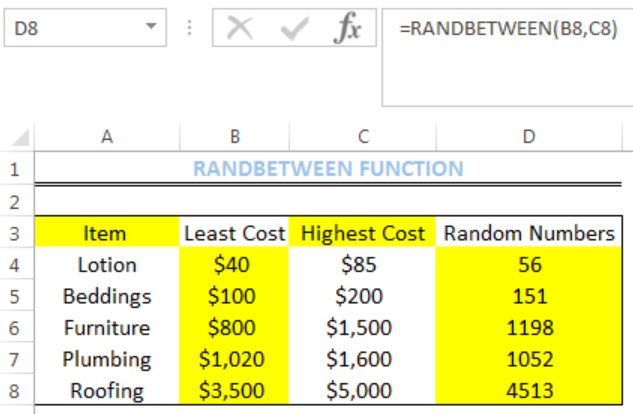Get instant live expert help with Excel or Google Sheets“My Excelchat expert helped me in less than 20 minutes, saving me what would have been 5 hours of work!”

#### Post your problem and you'll get expert help in seconds

Your message must be at least 40 characters
Our professional experts are available now. Your privacy is guaranteed.

# How to Use the Excel RANDBETWEEN Function

We can use the excel RANDBETWEEN function to generate random numbers in excel. The steps below will walk through the process.Figure 1: Result of RANDBETWEEN function for Cells of Column D

## General Formula

`=RANDBETWEEN(bottom,top)`

• Bottom – This is the smallest random integer that will be returned by the RANDBETWEEN function. It is the lowest value in the range.
• Top – This is the largest random integer that will be returned by the RANDBETWEEN function. It is the highest value in the range.

## Formula

`=RANDBETWEEN(B4,C4)`

## Setting up the Data

• We will set up the data by inputting the LEAST COST and HIGHEST COST into Column B and Column C respectively
• Column D is where we want the formula to return the result for the random numbersFigure 2: Setting up the Data

## Getting the Random Numbers

• We will click on Cell D4
• We will insert the formula below into the cell

`=RANDBETWEEN(B4,C4)`

• We will press the enter keyFigure 3: Result of RANDBETWEEN function for Cell D4

• We will click on Cell D4 again
• We will double-click on the fill handle (the small plus sign at the bottom right of Cell D4) and drag down to copy the formula into the other cellsFigure 4: Result of RANDBETWEEN function for Cells of Column D

## Notes

• RANDBETWEEN function generates a new random number if you click on an existing cell with the formula
• RANDBETWEEN function generates a new random number after every recalculation
• To retain random numbers, press the F9 button after using the RANDBETWEEN function in the cell.

## Instant Connection to an Expert through our Excelchat Service

Most of the time, the problem you will need to solve will be more complex than a simple application of a formula or function. If you want to save hours of research and frustration, try our live Excelchat service! Our Excel Experts are available 24/7 to answer any Excel question you may have. We guarantee a connection within 30 seconds and a customized solution within 20 minutes.

### Did this post not answer your question? Get a solution from connecting with the expert.Another blog reader asked this question today on Excelchat:## Subscribe to Excelchat.coAnother blog reader asked this question today on Excelchat: